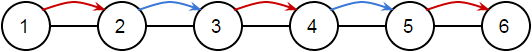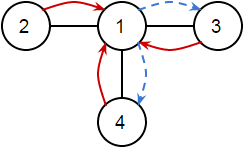시간 제한 메모리 제한 제출 정답 맞은 사람 정답 비율
2 초 256 MB 2 2 2 100.000%

## 문제

You are given a simple connected undirected graph with $N$ vertices and $M$ edges. The $i$-th edge connects the vertices $a_i$ and $b_i$.

Initially, the edges are not colored. Takahashikun wants to color the $i$-th edge with the color $c_i$.

He can color the edges in the following way:

• First he chooses a vertex, and he repeats zero or more steps.
• In each step, he chooses a vertex adjacent to the current vertex and moves to the chosen vertex along an edge. This edge is colored red or blue (the color is defined according to the rule below).
• In odd-indexed (1-based) steps he uses red. In even-indexed steps he uses blue.
• If he colors an already-colored edges, the color of the edge is updated to the new color.

Determine if he can color all edges with correct colors.

## 입력

$N$ $M$
$a_1$ $b_1$ $c_1$
$a_2$ $b_2$ $c_2$
$\vdots$
$a_M$ $b_M$ $c_M$

## 출력

Print "Yes", if Takahashikun can color all edges with correct colors or "No" otherwise.

## 제한

• $2 \leq N \leq 2000$
• $1 \leq M \leq 2000$
• $1 \leq a_i < b_i \leq N$
• The pairs $(a_i, b_i)$ are pairwise distinct.
• $c_i$ is either an 'r' (red) or a 'b' (blue).
• The graph is connected.

## 예제 입력 1

6 5
1 2 r
2 3 b
3 4 r
4 5 b
5 6 r


## 예제 출력 1

YesStart from vertex 1.

## 예제 입력 2

4 3
1 2 r
1 3 r
1 4 r


## 예제 출력 2

YesStart from vertex 2.

Dashed lines represent overpainted colors.

## 예제 입력 3

3 3
1 2 b
1 3 b
2 3 b


## 예제 출력 3

No